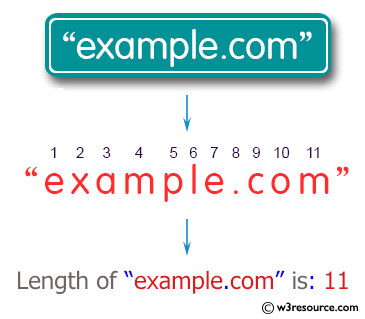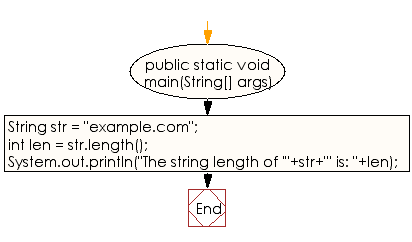﻿ Java exercises: Get the length of a given string - w3resource# Java String Exercises: Get the length of a given string

## Java String: Exercise-22 with Solution

Write a java program to get the length of a given string.

Pictorial Presentation:Sample Solution:

Java Code:

``````public class Exercise22 {
public static void main(String[] args)
{
String str = "example.com";
// Get the length of str.
int len = str.length();
System.out.println("The string length of '"+str+"' is: "+len);
}
}
```
```

Sample Output:

```The string length of 'example.com' is: 11
```

Flowchart:Java Code Editor:

Improve this sample solution and post your code through Disqus

What is the difficulty level of this exercise?

﻿

New Content: Composer: Dependency manager for PHP, R Programming